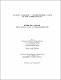## Exploiting the probability of observation for efficient Bayesian network inference2013
##### Authors
Mousumi, Fouzia Ashraf
##### Publisher
Lethbridge, Alta. : University of Lethbridge, Dept. of Mathematics and Computer Science
##### Abstract
It is well-known that the observation of a variable in a Bayesian network can affect the effective connectivity of the network, which in turn affects the efficiency of inference. Unfortunately, the observed variables may not be known until runtime, which limits the amount of compile-time optimization that can be done in this regard. This thesis considers how to improve inference when users know the likelihood of a variable being observed. It demonstrates how these probabilities of observation can be exploited to improve existing heuristics for choosing elimination orderings for inference. Empirical tests over a set of benchmark networks using the Variable Elimination algorithm show reductions of up to 50% and 70% in multiplications and summations, as well as runtime reductions of up to 55%. Similarly, tests using the Elimination Tree algorithm show reductions by as much as 64%, 55%, and 50% in recursive calls, total cache size, and runtime, respectively.
##### Description
xi, 88 leaves : ill. ; 29 cm
##### Keywords
Bayesian statistical decision theory -- Data processing , Elimination , Decision making -- Mathematical models , Dissertations, Academic